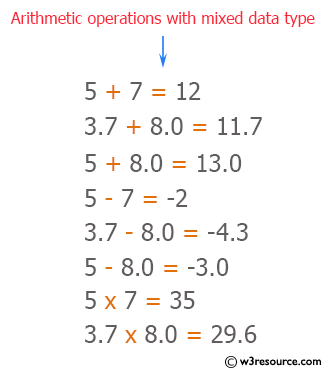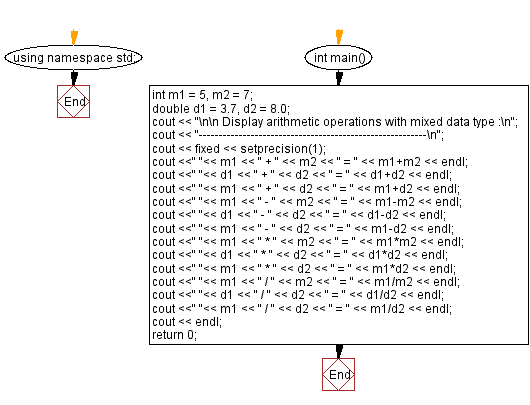﻿ C++ : Display various type or arithmetic operation

# C++ Exercises: Display various type or arithmetic operation using mixed data type

## C++ Basic: Exercise-7 with Solution

Write a C++ program that displays mixed data types and arithmetic operations.

Pictorial Presentation:Sample Solution:

C++ Code :

``````#include <iostream>
#include <iomanip>   // formatting floating-point numbers with 1 decimal place
using namespace std;

int main()
{
int m1 = 5, m2 = 7;
double d1 = 3.7, d2 = 8.0;

cout << "\n\n Display arithmetic operations with mixed data type :\n";
cout << "---------------------------------------------------------\n";
cout << fixed << setprecision(1);

cout <<" "<< m1 << " + " << m2 << " = " << m1+m2 << endl;
cout <<" "<< d1 << " + " << d2 << " = " << d1+d2 << endl;
cout <<" "<< m1 << " + " << d2 << " = " << m1+d2 << endl;

cout <<" "<< m1 << " - " << m2 << " = " << m1-m2 << endl;
cout <<" "<< d1 << " - " << d2 << " = " << d1-d2 << endl;
cout <<" "<< m1 << " - " << d2 << " = " << m1-d2 << endl;

cout <<" "<< m1 << " * " << m2 << " = " << m1*m2 << endl;
cout <<" "<< d1 << " * " << d2 << " = " << d1*d2 << endl;
cout <<" "<< m1 << " * " << d2 << " = " << m1*d2 << endl;

cout <<" "<< m1 << " / " << m2 << " = " << m1/m2 << endl;
cout <<" "<< d1 << " / " << d2 << " = " << d1/d2 << endl;
cout <<" "<< m1 << " / " << d2 << " = " << m1/d2 << endl;
cout << endl;
return 0;
}
``````

Sample Output:

``` Display arithmetic operations with mixed data type :
---------------------------------------------------------
5 + 7 = 12
3.7 + 8.0 = 11.7
5 + 8.0 = 13.0
5 - 7 = -2
3.7 - 8.0 = -4.3
5 - 8.0 = -3.0
5 * 7 = 35
3.7 * 8.0 = 29.6
5 * 8.0 = 40.0
5 / 7 = 0
3.7 / 8.0 = 0.5
5 / 8.0 = 0.6
```

Flowchart:C++ Code Editor:

What is the difficulty level of this exercise?

﻿

## C++ Programming: Tips of the Day

Why is there no std::stou?

The most pat answer would be that the C library has no corresponding "strtou", and the C++11 string functions are all just thinly veiled wrappers around the C library functions: The std::sto* functions mirror strto*, and the std::to_string functions use sprintf.

Ref: https://bit.ly/3wtz2qA

We are closing our Disqus commenting system for some maintenanace issues. You may write to us at reach[at]yahoo[dot]com or visit us at Facebook